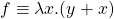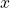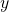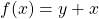# Using Dart’s C-style syntax to make chain of lambda functions concrete

Start with an example lambda calculus expression. It is:

• [programming view] a function withas an input argument and it usesfrom the outer workspace (called a free-variable). f(var x) { return y+x; }
• [mathematical view] can also be written aswhereis seen as a fixed/snapshotted value relative to the expression.
g(int y) => (int x) => y + x

Lambda calculus is right-associative

g(int y) => ( (int x) => y + x )

Unrolling in C-style it will give better insights to the relationships between layers

g(int y) {
return (int x) { return y + x; };
}

Note that (int x) { return y + x; } is a functor. To emphasize this, the same code can be rewritten by assigning the name f to it and returning f:

g(int y) {
f(int x) { return y + x; };
return f;
}

Use the C++11 style syntax so that it doesn’t look like nested function implementation body instead of a functor nested inside a function:

g(int y) {
var f = (int x) { return y + x; };
return f;
}

Note that conceptually what we are doing with the wrapper cascade is indeed nested functions. However, in the wrapper, we spit out a functor (which did most of the partial work) so the user can endow/evaluating it with the last piece of needed info/input:

g(int y) {
f(int x) {
return y + x;
};
return f;
}

More commonly as seen in Dart docs, this formatting shows a (binder/capturing) wrapper function returning its own nested function:

g(int y) {
return (int x) {
return y + x;
};
}

20 total views,  1 views today

Subscribe
Notify of